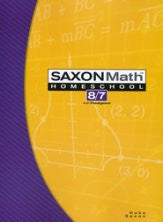# Math 8/7 Homeschool Student Edition (3rd Edition)

• \$ 56.80

Reviews arithmetic calculation, measurements, geometry and other skills, and introduces pre-algebra, ratios, probability and statistics. Students will specifically learn about adding/subtracting/multiplying fractions, equivalent fractions, the metric system, repeating decimals, scientific notation, Pi, graphing inequalities, multiplying algebraic terms, the Pythagorean Theorem, the slope-intercept form of linear equations, and more .

931 indexed pages, consumable, softcover. Reproduction of most pages is prohibited.